# Hexahedron

A hexahedron (plural: hexahedra) is any polyhedron with six faces. A cube, for example, is a regular hexahedron with all its faces square, and three squares around each vertex.

There are seven topologically distinct convex hexahedra, one of which exists in two mirror image forms. (Two polyhedra are "topologically distinct" if they have intrinsically different arrangements of faces and vertices, such that it is impossible to distort one into the other simply by changing the lengths of edges or the angles between edges or faces.)

Quadrilaterally-faced hexahedron (Cuboid) 6 faces, 12 edges, 8 vertices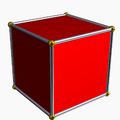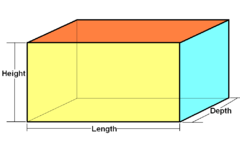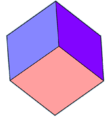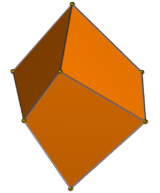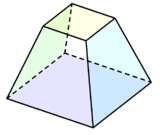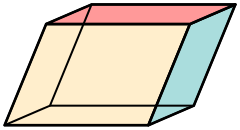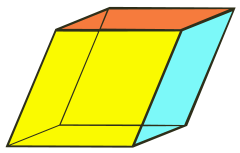Cube
(square)
Rectangular cuboid
(three pairs of
rectangles)
Trigonal trapezohedron
(congruent rhombi)
Trigonal trapezohedron
(apex-truncated
square pyramid)
Parallelepiped
(three pairs of
parallelograms)
(three pairs of
rhombi)
Oh, [4,3], (*432)
order 48
D2h, [2,2], (*222)
order 8
D3d, [2+,6], (2*3)
order 12
D3, [2,3]+, (223)
order 6
C4v, , (*44)
order 8
Ci, [2+,2+], (×)
order 2Triangular bipyramid36 Faces9 E, 5 VTetragonal antiwedge. Chiral – exists in "left-handed" and "right-handed" mirror image forms.4.4.3.3.3.3 Faces10 E, 6 V4.4.4.4.3.3 Faces11 E, 7 VPentagonal pyramid5.35 Faces10 E, 6 V5.4.4.3.3.3 Faces11 E, 7 V5.5.4.4.3.3 Faces12 E, 8 V

There are three further topologically distinct hexahedra that can only be realised as concave figures:

Concave4.4.3.3.3.3 Faces
10 E, 6 V5.5.3.3.3.3 Faces
11 E, 7 V6.6.3.3.3.3 Faces
12 E, 8 V

## References

1. Counting polyhedra